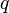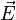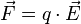# Electric field facts for kids

Kids Encyclopedia Facts

An electric field is a vector field that shows the direction that a positively charged particle will move when placed in the field. More precisely, if a particle has an electric charge$q$ and is in an electric field$\vec{E}$, the electric force the charge will feel is$\vec{F}=q\cdot \vec{E}$ .Electric fields are produced around objects that have electrical charge, or by a magnetic field that changes with time. Electric field lines are used to represent the influence of electric field. The idea of an electric field was first made by Michael Faraday.

Electric fields are caused by electric charges, described by Gauss's law, or varying magnetic fields, described by Faraday's law of induction. The equations of both fields are coupled and together form Maxwell's equations that describe both fields as a function of charges and currents.Electric field Facts for Kids. Kiddle Encyclopedia.